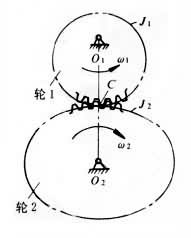# Non-circular Gear

Non-circular gears are also called special-shaped gears, which are gears whose indexing surface is not a rotating surface.After it and the other gear make up the gear pair, during the meshing process, the instantaneous angular velocity ratio changes according to a certain motion law.

## 1) The characteristics of non-circular gears

Non-circular gears can achieve special motion and function calculations, which are beneficial to the motion characteristics of the mechanism.

It can improve the performance of the mechanism and improve the movement conditions of the mechanism.

## 2) The application

Non-circular gears are widely used in automatic machine instrumentation and solving devices.

## 3) Non-circular gear transmission

Non-circular gear transmission refers to a gear transmission in which the section surface of at least one of the gears is not a rotating curved surface.

The section surface of at least one of the gears in the transmission is not a geared surface of a rotating surface.

When a pair of non-circular gears meshes with the transmission, the instantaneous transmission ratio changes periodically according to a certain regularity.The section curves of the two non-circular gears 1 and 2 in Fig. 1 are J1 and J2, respectively.

When the two-wheel meshing drive, J1 and J2 make a pure roll without slip.

Its instantaneous transmission ratio is: i12 = ω1 / ω2 = r2 / r1.

Where ω1 and ω2 are the instantaneous angular velocities of the two rounds, respectively, and r1 and r2 are the instantaneous vector radii of the two rounds, respectively.

In order to achieve non-circular gear transmission, that is, to ensure that the two-wheel instantaneous trajectory is a pure rolling contact, the following two conditions must be met:

① The sum of any two instantaneous vector radii should be equal to the center distance a of the two rounds, ie r1 + r2 = a;

② The length of the two-section curve J2 must be an integral multiple of J1.

There are many types of non-circular gear transmissions, and elliptical gear transmissions are commonly used in machinery.

Its section curve is a pair of ellipticals of equal size.

In the elliptical gear transmission, when the driving wheel rotates at a constant speed, the angular velocity of the driven wheel periodically changes from minimum to maximum, and from maximum to minimum.

In addition, other curves can be used as section curves, such as logarithmic spirals and oval curves.

Non-circular gear drives are used in machine tools, printing presses, textile machinery and instruments.

Maybe you ...

## Need a Quote? Have Questions?